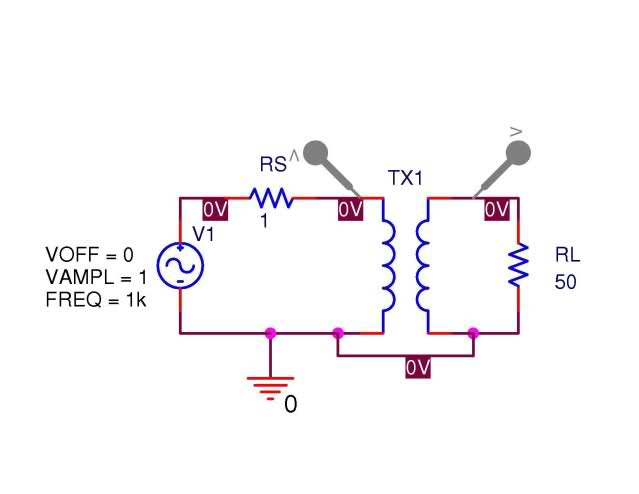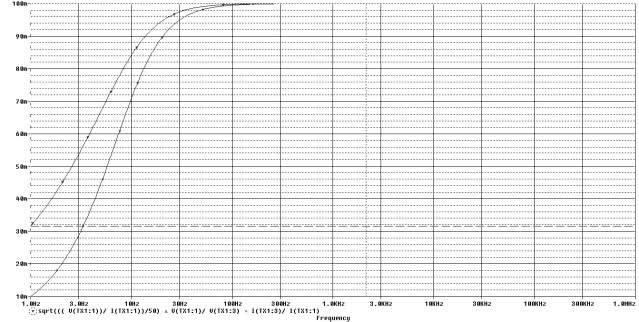# Working Range of Transformer

AIR&SPACE

## Homework Statement

By trying a variety of values for $$L_{S}$$ and $$L_{L}$$ find a transformer for which the three relationships are satisfied while, a=10. Use smallest possible inductances you need to make the transformer work properly @ 1kHz and above. Print plot with the three ratios that demonstrate the transformer works at range specified. Repeat this process so that the transformer works at 100kHz and up.
It is given that the coupling coefficient is 1

## Homework Equations

$$Z_{in}$$=$$\frac{R_{L}}{a^{2}}$$

a=$$\frac{V_{L}}{V_{S}}$$

a=$$\frac{I_{S}}{I_{L}}$$Problem statement goes on to tell me to produce a graph using these equations as traces, note that they want me to graph 1/a instead of a.

$$\frac{1}{a}$$=$$\frac{V_{L}}{V_{S}}$$

$$\frac{1}{a}$$=$$\frac{I_{L}}{I_{S}}$$

$$\frac{1}{a}$$=$$\sqrt{\frac{1}{50}\frac{V_{S}}{I_{S}}}$$

## The Attempt at a Solution

So really, my question boils down to this $$\Rightarrow$$ How is the working frequency of this circuit defined? Here is a graph I made with 8mH and 800mH respectively.First eqn is straight line @ 100m, second eqn is bottom curved line, 3rd is the middle line.

Now I know (or at least I think I do) that by "work" they mean where it acts as a high-pass filter, since that is what this is. My "real world" insight tells me that the two curved lines will never hit 100m. But, in this circuit we assumed a perfect coupling coefficient. Does that mean these lines actually do intersect? If they do, I would assume that is what is being looked for. Otherwise, I would assume there is one of two things at work.
1) A ratio of $$\frac{Z_{out}}{Z_{in}}$$ that defines when this circuit works. If this is the case, what is this ratio?
2) It has something to do with the resonant (corner) frequency of the filter, for an RL Circuit $$f_{c}$$=$$\frac{R}{2\pi L}$$ But this, to me, wouldn't account for the mutual inductance.

I would prefer insight or direction as opposed to formula's or a road map. But I won't complain. Thanks in advance.

P.S. For those curious, circuit designed in Orcad Capture, simulated in Orcad PSpice, class is Electronic Instrumentation @ RPI

$$\frac{1}{\sqrt{2}}\approx\frac{1}{\sqrt{1+\left(\frac{R_{pa}}{2*\pi*f*L_{oc}}\right)^{2}}}$$
Where $$R_{pa}$$ is the resistance there would be if the resistors were in parallel ; $$L_{oc}$$ is the Inductor's open circuit inductance and $$f$$ is frequency in Hz.